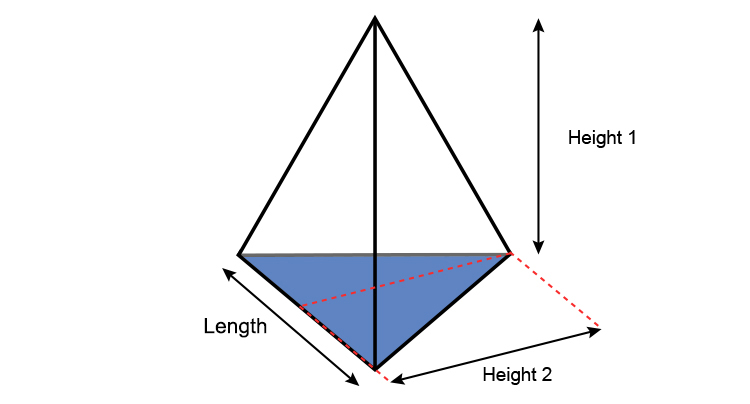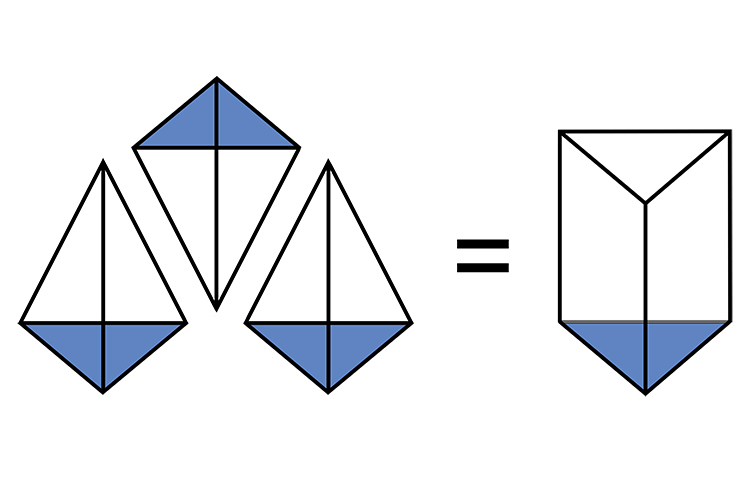# Volume of triangular based pyramids

The volume of a triangular based pyramid works on the area of the base multiplied by height and some factor.Base area of triangular based pyramid = 1/2times\l\e\n\g\t\h\times\height\ 2

Volume of a triangular based pyramid = 1/3times\base\ area\times\height\ 1

Volume of a triangular based pyramid = 1/3times(1/2times\base\times\height\ 2)times\height\ 13 triangular based pyramids fill 1 prism (with the same size base and height 1)

NOTE:

Areas shaded blue are all equal.